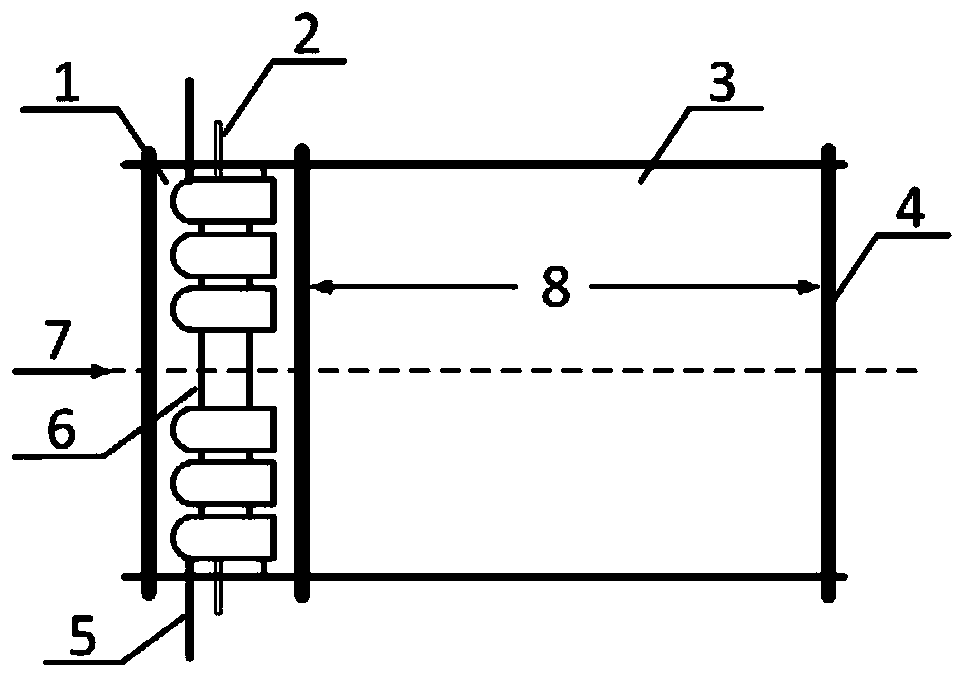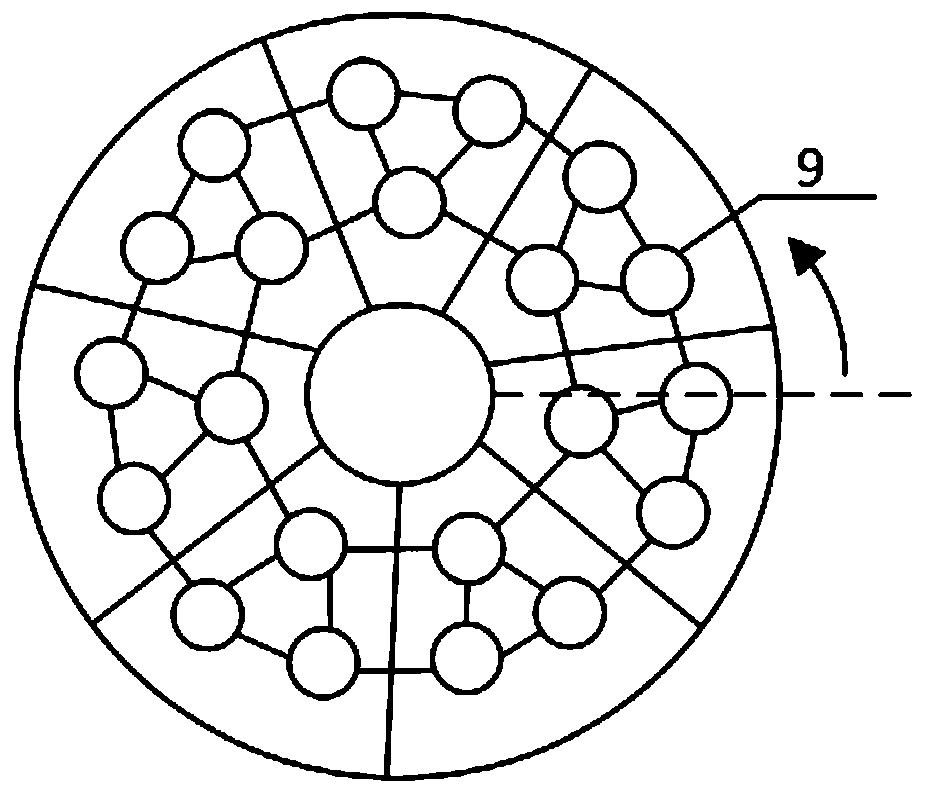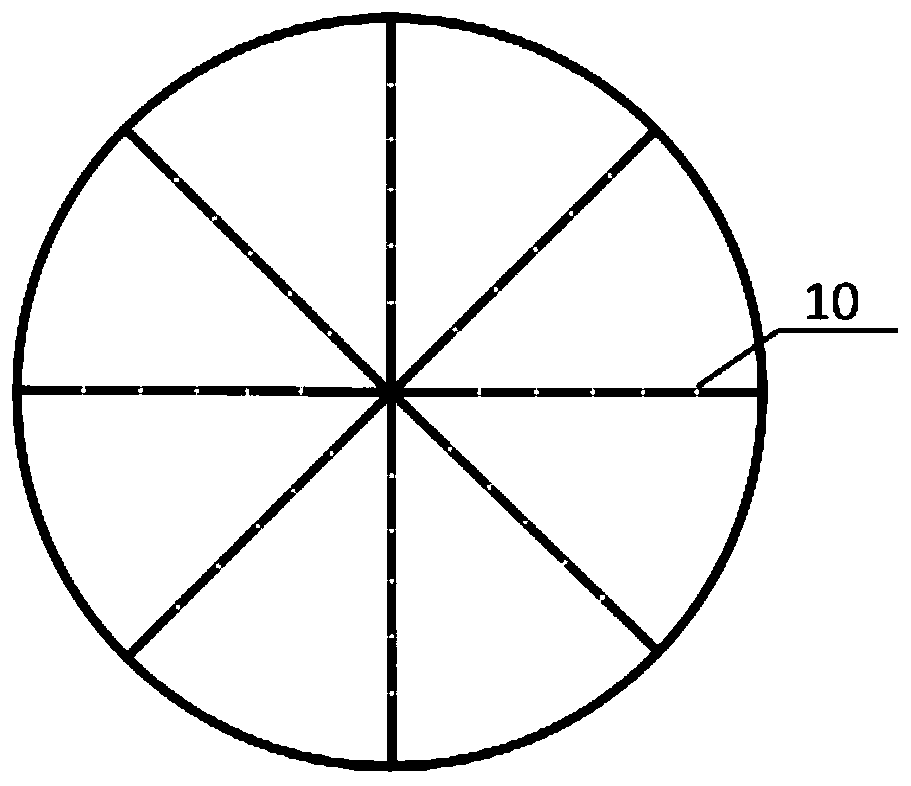# Temperature distortion spectrum inverse design method based on discrete sequence

## A discrete sequence and anti-design technology, applied in computer-aided design, design optimization/simulation, calculation, etc., to shorten the test cycle, avoid waste of manpower and material resources, and reduce test costs

Active Publication Date: 2019-08-02
XIAMEN UNIV
3 Cites 2 Cited by

## AI-Extracted Technical Summary

### Problems solved by technology

 The purpose of the present invention is to aim at the deficiencies of the existing accurate design method of temperature distortion map, calculate the he...
View more

## Abstract

The invention discloses a temperature distortion spectrum inverse design method based on a discrete sequence. The method comprises steps of determining the incoming flow speed and the distance betweenthe upstream distortion section and the downstream map section; numbering nozzle arranging distributing, and setting a heat flow combination sequence of each nozzle as a vector; dividing a downstreamtarget map cross section into units of magnitude N respectively, carrying out micro-unit sorting outwards on the divided micro-units by taking the horizontal direction as a zero angle line, and recording the micro-units as map column vectors beta; respectively constructing column vectors Q1,..., Qi,... and QM, and measuring a downstream temperature distortion spectrum; taking a generalized inverse matrix B for the matrix A, and determining the distribution of heat flow; according to the vector alpha, obtaining the state of each upstream nozzle. The specific temperature distortion spectrum isachieved by adjusting the heat flow injection intensity, the obtained result provides data support for exploring the temperature distortion under the real working condition, and therefore the inversedesign method is that a matrix A and the column vector beta are used for inversely solving the column vector alpha.

Application Domain

Technology Topic

DistortionColumn vector +6

## Image

•••## Examples

• Experimental program(1)

### Example Embodiment

 The following embodiments will further illustrate the present invention in conjunction with the drawings.
 The embodiment of the present invention includes the following steps:
 1) Determine the incoming flow velocity and the distance between the upstream distortion section and the downstream profile section according to the given temperature profile distribution of the measurement section;
 2) Generate nozzle number M and nozzle distribution according to temperature, number the arrangement and distribution of nozzles sequentially, and set the heat flow combination sequence of each nozzle as vector α;
 3) Divide the downstream target map cross-section into units of magnitude N, and sort the minute units with the horizontal direction as a zero-angle line, circle and circle outward, and use interpolation based on the temperature distortion map Assign temperature to N units in the way of, and record it as the map column vector β;
 4) Construct the column vector Q separately 1 ,...,Q i ,...,Q M , Where Q i Denoted as (q 1 ,...,Q i ,...,Q M ) T , Except q 1 Except for 1, the other values ​​are set to 0, which means that except the i-th nozzle is in the maximum heat flow state, the other M-1 nozzles are in the closed state, where the maximum heat flow state is recorded as 1, and the minimum heat flow state is recorded as 0;
 5) Carry out numerical simulation or experimental research respectively in which the i-th nozzle is at the maximum heat flow and the other M-1 are in the closed state, measure the downstream temperature distortion map, and refer to the number of step 3) to obtain the vector (a 1i , A 2i , A 3i …A Ni ) T , A total of M nozzles form a matrix
 6) According to Aα=β, take the generalized inverse matrix B for matrix A, where BA=E M , E M Is the M-order unit matrix, and reverse the heat flow distribution vector α, that is, α=Bβ, so as to determine the heat flow distribution, where the maximum value 1 and the minimum value 0 in the vector α;
 7) According to the obtained vector α, the status of each upstream nozzle is obtained, that is, the specific temperature distortion map is realized by adjusting the heat flow injection intensity, and the obtained result provides data support for exploring the temperature distortion under real working conditions. Therefore, this inverse design method is Use matrix A and column vector β to inversely find column vector α.
 figure 1 A schematic top view of the temperature distortion test device is given. It is roughly the same as the traditional test device. The ignition nozzle 9 is mutually stable by the fixed rod 6 in the combustion section 1 in the test section, and the fuel pipe 2 in the combustion section 1 in the test section and The gas pipe 5 in the combustion section 1 of the test section communicates with each nozzle; the length of the mixing section 3 in the test section is the ignition nozzle in the test section from the cross section of the ignition nozzle 9 to the temperature measurement surface 4 downstream of the test section Distance from cross section to temperature measuring surface 8. The heat flow distribution deduced by the inverse design method is mainly manifested in figure 2 On the cross section of the ignition nozzle, adjust the ignition nozzle 9 in the combustion section 1 in the test section according to the result vector α of the inverse design method, and use the temperature measurement point 10 in the temperature measurement surface on the temperature measurement surface 4 downstream of the test section to obtain Data, obtained after processing the data Figure 4 The temperature distortion simulation map is compared with the known temperature distortion map. If the closeness is good, the simulated heat flow field can represent the real heat flow field.
 The temperature distortion test section of the present invention has two main sections, namely the upstream cross section of the ignition nozzle and the downstream temperature measurement surface. In actual operation, according to the given temperature distortion map, the temperature value of each unit is determined on the temperature measurement surface, and then the heat flow distribution in the real working condition is simulated according to the inverse design method, as follows:
 Definition: the combustion intensity of the i-th ignition nozzle is α i , The heat flow distribution is obtained as the vector α; the temperature value obtained at each measuring point on the downstream temperature measuring surface is recorded as b j , All the temperature values ​​constitute the map column vector β.
 Suppose the heat flow distribution at a certain moment is α=(α 1 ,α 2 ,...,α i ,...,Α M ) T , And the temperature of a certain measuring point j on the temperature measuring surface at that moment is affected by each nozzle based on other factors except heat flow as α j1 ,α j2 ,...,α ji ,...,Α jM , These values ​​are measured from experiments, in which only the influence of the three ignition nozzles on a certain axis on the downstream temperature measuring surface can be calculated to obtain the values ​​of the remaining nozzles. According to the principle of linear superposition of data points, the influence of point j under other factors is α j1 +α j2 +α ji +…+α jM , Before each term of the above formula, multiply by the combustion intensity α of each nozzle at this time i We can get the total effect of temperature at that moment n b j , Which is b j =α 1 *α j1 +α 2 *α j2 +α i *α ji +…+α M *α jM. Therefore, according to this idea and so on, matrix A can be constructed to reflect the mapping law of the temperature influence of the upstream heat source on the downstream temperature measurement surface:
 And Aα=β
 According to Aα=β, take the generalized inverse matrix B for matrix A, where BA=E M , E M It is an M-order unit matrix, so the heat flow distribution vector α can be reversed, that is, α=Bβ, so as to determine the heat flow distribution.
 The invention avoids the waste of manpower and material resources caused by a large number of random attempts in the experiment, and greatly shortens the test cycle; at the same time, the reverse design method only needs to establish a database of matrix A with the mapping law, and can obtain different temperature distortion maps The corresponding heat flow distribution can be obtained quickly, which significantly reduces the test cost compared with the traditional method.
 Such as Figure 1~4 As shown, the present invention includes the combustion section in the test section 1, the fuel pipe in the combustion section 1 in the test section, the mixing section 3 in the test section, the temperature measurement surface 4 downstream of the test section, and the combustion section in the test section The gas pipe in 1 5, the fixed rod in the combustion section 1 in the test section, the air inlet in the upstream of the test section 7, the distance from the cross section of the ignition nozzle in the test section to the temperature measurement surface, the ignition nozzle 9, the temperature measurement The temperature measurement point 10 in the surface and the high temperature distortion area 11 in the temperature distortion map.
 First, determine the distance from the cross section of the ignition nozzle to the temperature measurement surface according to the engine model. Then, according to the working state of the engine, the 12 ignition nozzles 9 are sequentially numbered according to the arrangement and distribution, and the combustion intensity function curve of each ignition nozzle 9 is calculated.
 The numbering rules are as follows:
 On the circular section of the heat source, with the center of the circle as the vertex, a ray is drawn horizontally to the right. This ray is set as the horizontal reference line, that is, the 0° line, and the counterclockwise rotation is positive. Rotate the horizontal reference line in the forward direction and find the ignition nozzles. The ignition nozzles 9 on the same ray are numbered radially outward from the center of the circle. According to the above numbering method, the M ignition nozzles 9 are sorted in sequence.
 The combustion intensity of each ignition nozzle is obtained by adjusting the gas and oil supply. Theoretically, the maximum combustion intensity is set to 1, the minimum is 0, and the remaining values ​​are obtained between 0 and 1.
 Divide the downstream target map section into units of magnitude N, and sort the minute units with a zero-angle line in the horizontal direction, round and round outwards, and use interpolation based on the temperature distortion map Assign temperature values ​​to N units and record them as map column vector β.
 Control the operation of the i-th ignition nozzle, leaving M-1 ignition nozzles closed, namely Q i =(q 1 ,...,Q i ,...,Q M ) T , Where q i Set to 1, other values ​​are set to 0, and the maximum heat flow state is recorded as 1, and the minimum heat flow state is recorded as 0. Carry out numerical simulation or experimental research respectively in which the i-th ignition nozzle is at the maximum heat flow and the other M-1 are in the closed state, measure the downstream temperature distortion map, and refer to the numbering rule of the above-mentioned downstream target map section to obtain the vector (α 1i ,α 2i ,...,α 3i ,...,Α Ni ) T. A total of M ignition nozzles 9 can form a matrix
 According to Aα=β, take the generalized inverse matrix B for matrix A, where BA=E M , E M Is the M-order unit matrix. Therefore, the heat flow distribution vector α can be inversely calculated, that is, α=Bβ, and the combustion intensity curve obtained by fitting discrete points can be calculated to obtain the current gas delivery and fuel supply state of each ignition nozzle 9, that is, the current heat flow distribution.
 The heat flow distribution obtained by the above inverse design method is inversely deduced and obtained after the simulation test Figure 4 The temperature distortion map is compared with the known temperature distortion map, especially the area and orientation of the high temperature distortion zone 11 to simulate the true heat flow distribution. The invention avoids the waste of manpower and material resources caused by a large number of random attempts in the experiment, and greatly shortens the test cycle; at the same time, the reverse design method only needs to establish a database of matrix A with the mapping law, and can obtain different temperature distortion maps The corresponding heat flow distribution can be obtained quickly, which significantly reduces the test cost compared with the traditional method.

## PUM## Description & Claims & Application Information

We can also present the details of the Description, Claims and Application information to help users get a comprehensive understanding of the technical details of the patent, such as background art, summary of invention, brief description of drawings, description of embodiments, and other original content. On the other hand, users can also determine the specific scope of protection of the technology through the list of claims; as well as understand the changes in the life cycle of the technology with the presentation of the patent timeline. Login to view more.

## Light storage and charging control system and method

PendingCN114884128AAvoid wasting manpower and material resourcesAvoid shorting the power supply
Owner:绿能慧充数字技术有限公司

## A new energy vehicle vehicle control strategy and network test platform

Owner:SAIC-GM-WULING AUTOMOBILE CO LTD

## A half clasp fatigue test assembly and fatigue test device

Owner:XIAN AVIATION BRAKE TECH

## Simulation method and system based on ADAMS vehicle double-shift-line test

Owner:JIANGLING MOTORS

## Interlayer alignment visual monitoring method

InactiveCN112165854AAvoid wasting manpower and material resourceslow cost
Owner:YIXING SILICON VALLEY ELECTRONICS TECH

## Cabin filling method and cart for cabin filling device

ActiveCN109279046Aavoid manual handlingShorten the test cycle
Owner:CHINA AIRPLANT STRENGTH RES INST

## Variable-speed pumped storage unit rotor short circuit and eccentricity fault diagnosis method

PendingCN114492597AAvoid wasting manpower and material resources
Owner:HUAZHONG UNIV OF SCI & TECH

## Horizontal table top capable of switching multiple stations and horizontal vibration testing machine

PendingCN113109009ASave test man-hour costShorten the test cycle
Owner:SUZHOU SUSHI TESTING INSTR CO LTD

## Classification and recommendation of technical efficacy words

• Shorten the test cycle
• Avoid wasting manpower and material resources

## Load spectrum simplification method for aircraft construction crack extension test

Owner:CHINA AIRPLANT STRENGTH RES INST

## Friction load coupling experimental device and friction load coupling experimental method in marine climate environment

Owner:UNIV OF SCI & TECH BEIJING +1

## Simulated large-field soil and solute transporting system

InactiveCN102608291AShorten the test cycleReduce boundary effects
Owner:CHINA AGRI UNIV

## Interface automatic testing method and device for vehicle control unit

Owner:BEIJING ELECTRIC VEHICLE

## Laser cladding bonding-based cutter and workpiece diffusion couple making method

Owner:SHANDONG UNIV
Who we serve
• R&D Engineer
• R&D Manager
• IP Professional
Why Eureka